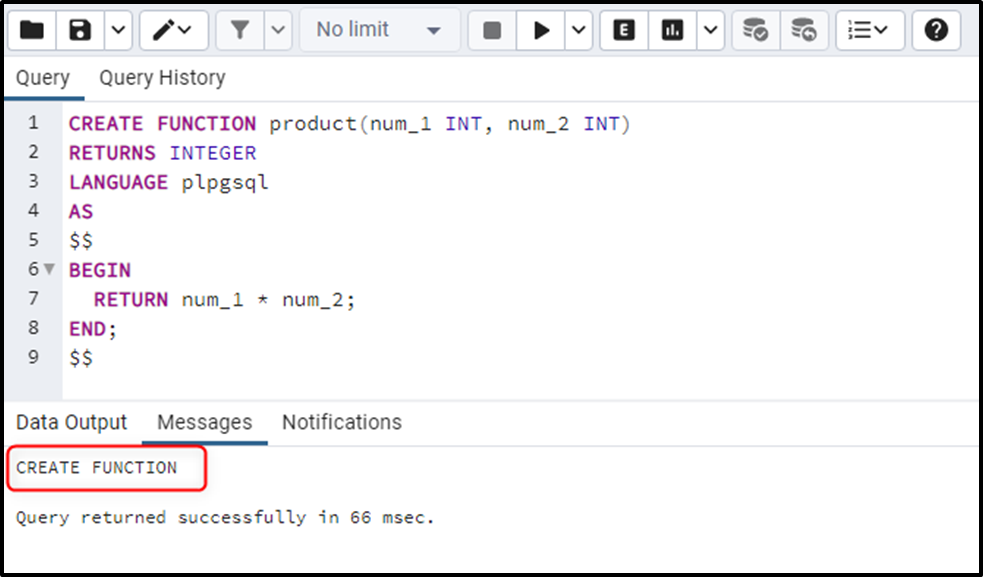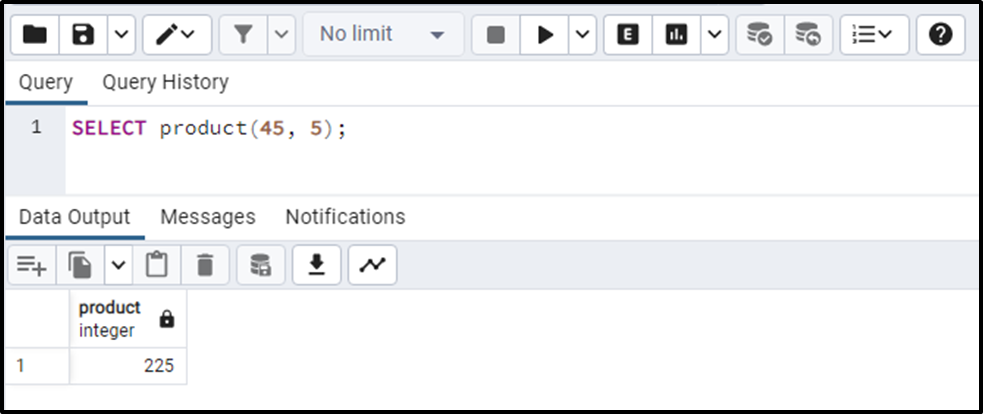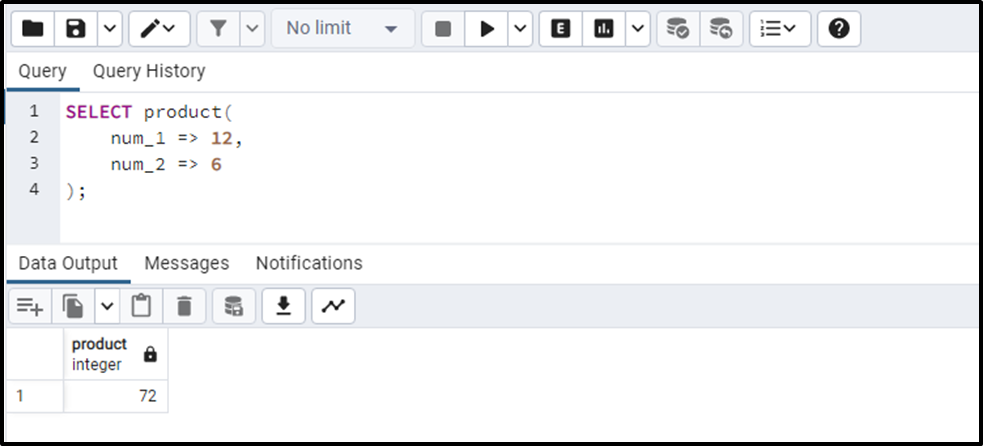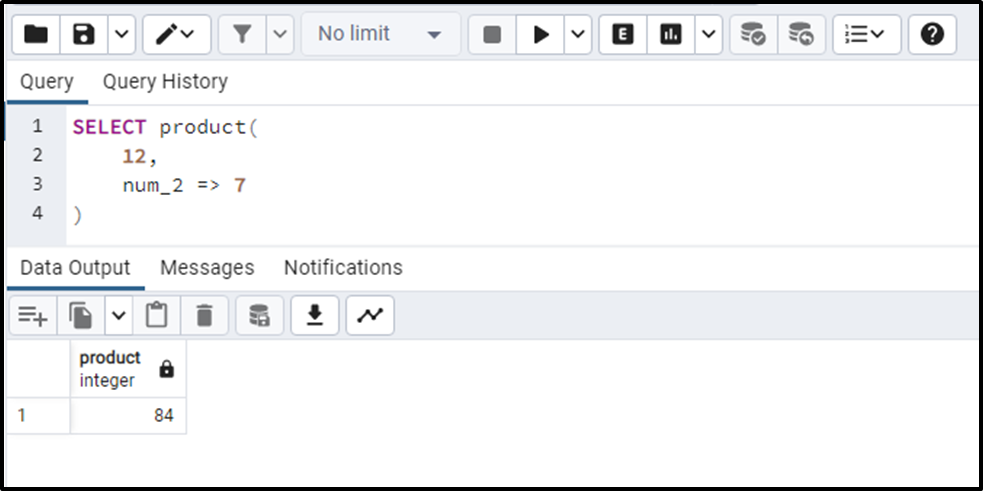##### How to Call a User-defined Function in PostgreSQL

In PostgreSQL, user-defined functions allow us to execute a group of statements to get the desired results. Using Postgres functions, we can create custom logic and reutilize it in our database when needed. To utilize the functionality of a user-defined function in PostgreSQL, we need to invoke/call it. In this write-up, we will illustrate different methods to invoke a user-defined function in Postgres.

How to Call a User-defined Function in Postgres?

Calling/invoking a user-defined function requires the function name and its parameters(if any). Depending on how it is implemented, a user-defined function may retrieve a value or perform some specific actions. To call a user-defined function in Postgres, use one of the following methods:

• Positional Notation
• Named Notation
• Mixed notation

How to Call a User-defined Function Using Positional Notation?

To call a user-defined function using positional notation, all you need to do is specify the argument in the same order as the parameter’s order while calling a function. Here is the syntax to call a user-defined function using the positional notation:

`SELECT func_name(parameter_list);`

Example: Positional Notation

Let’s first create a function named “product” that accepts two numbers and retrieves their multiplication:

```CREATE FUNCTION product(num_1 INT, num_2 INT)
RETURNS INTEGER
LANGUAGE plpgsql
AS
\$\$
BEGIN
RETURN num_1 * num_2;
END;
\$\$```Let’s call the “product” function using the positional notation:

`SELECT product(45, 5);`The output shows that the stated function retrieves the product/multiplication of given numbers.

How to Call a User-defined Function Using Named Notation?

Calling a user-defined function with named notation allows us to specify the parameter values along with their names at the time of function invoking. It is recommended that the “Named Notation” approach should be used when there are many parameters as it eliminates ambiguity. The below snippet shows the basic syntax:

```SELECT func_name(
parameter_name_1 => val,
parameter_name_2 => val
);```

Example: Named Notation

In this example, we will utilize the same “product” function, however, we will invoke it using the named notation:

```SELECT product(
num_1 => 12,
num_2 => 6
);```The output proves that calling the product function with a named notation retrieves accurate results.

How to Call a User-defined Function Using Mixed Notation?

As the name itself suggests, Mixed Notation is a mixture of both “named notation” and “positional notation”. This means the Mixed notation approach allows us to call a function by specifying the parameters using both named and positional notations. However, the positional arguments must come first, and then comes the named arguments. Else you will experience an error. Here is a syntax to use the mixed notation:

```SELECT func_name(
parameter_val,
parameter_name => parameter_val
);```

Example: Mixed Notation

The below code snippet demonstrates the use of mixed notation in Postgres:

```SELECT product(
12,
num_2 => 6
);```That’s all about calling a user-defined function in Postgres using different notations.

Conclusion

To call a user-defined function in Postgres, Positional Notation, Named Notation, or Mixed Notation can be used. Calling/invoking a user-defined function requires the function name and its parameters(if any). Depending on how it is implemented, a user-defined function may retrieve a value or perform some specific actions. This write-up has illustrated various notations to call a user-defined function in PostgreSQL.